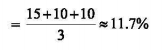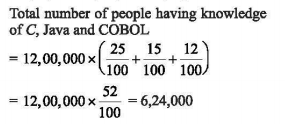# UGC NET Paper 1 December (Pack of 3) - UGC NET PAPER 1 2nd December 2019 Morning Shift

>>>>>>>>UGC NET PAPER 1 2nd December 2019 Morning Shift

The following table gives the percentage (%) of people having knowledge of different programming languages. Assuming that the total number of people having knowledge about programming language is 12, 00,000 answer the questions that follow the table:

 Programming Language % of People having knowledge C++ 15% C 25% COBOL 12% FORTRAN 13% Java 15% LISP 10% Python 10%

• A

1.04%• B

11.7%• C

2.66%• D

11.0%• Option : B
• Explanation : Average percentage (%) of people having programming language - python, LISP and JavaThe following table gives the percentage (%) of people having knowledge of different programming languages. Assuming that the total number of people having knowledge about the programming language is 12,00,000 answer the questions that follow the table:

• A

3: 5• B

5: 3• C

1: 1• D

5: 2• Option : B
• Explanation :The following table gives the percentage (%) of people having knowledge of different programming languages. Assuming that the total number of people having knowledge about the programming language is 12,00,000 answer the question that follows the table:

• A

1,20,000• B

1,80,000• C

1,44,000• D

1,56,000• Option : D
• Explanation : Number of people having knowledge of C
= 12,00,000 X 25/100 = 3,00,000
Number of people having knowledge of
Cobol
= 12,00,000 X 12/100 = 1,44,000
Difference in number of peoples
= 3,00,000 - 1,44,000 = 1,56,000

The following table gives the percentage (%) of people having knowledge of different programming languages. Assuming that the total number of people having knowledge about the programming language is 12,00,000 answer the question that follows the table:

• A

4,80,000• B

5,04,000• C

5,16,000• D

6,24,000• Option : D
• Explanation :The following table gives the percentage (%) of people having knowledge of different programming languages. Assuming that the total number of people having knowledge about the programming language is 12,00,000 answer the question that follows the table:

• A

1,80,000• B

3,00,000• C

1,56,000• D

1,44,000• Option : B
• Explanation : Number of people having knowledge about
'C' language
= 12,00,000 X 25/100 = 3,00,000